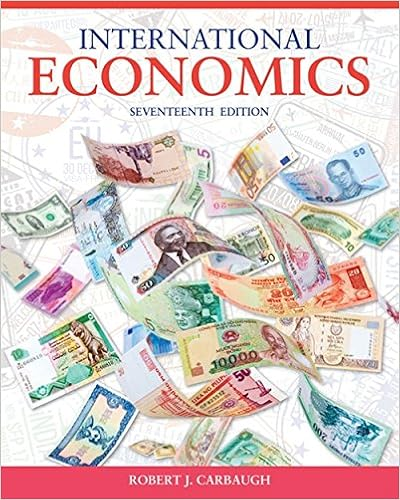# Draw the tree for a call option on 20000 with a

• bruiser143
• 43
• 93% (30) 28 out of 30 people found this document helpful

This preview shows page 25 - 29 out of 43 pages.

##### We have textbook solutions for you!
The document you are viewing contains questions related to this textbook.The document you are viewing contains questions related to this textbook.
Chapter 11 / Exercise 7
International Economics
CarbaughExpert Verified
59. Draw the tree for a call option on \$20,000 with a strike price of £10,000. The current exchange rate is £1.00 = \$2.00 and in one period the dollar value of the pound will either double or be cut in half. The current interest rates are i\$= 3% and are i£= 2%. A. B.C. None of the above
Topic: Binomial Option-Pricing Model7-25
##### We have textbook solutions for you!
The document you are viewing contains questions related to this textbook.The document you are viewing contains questions related to this textbook.
Chapter 11 / Exercise 7
International Economics
CarbaughExpert Verified
Chapter 07 - Futures and Options on Foreign Exchange60. Find the hedge ratio for a put option on \$15,000 with a strike price of €10,000. In one period the exchange rate (currently S(\$/€) = \$1.50/€) can increase by 60% or decrease by 37.5% (i.e.u= 1.6 and d= 0.625).
Topic: Binomial Option-Pricing Model61. Find the hedge ratio for a put option on €10,000 with a strike price of \$15,000. In one period the exchange rate (currently S(\$/€) = \$1.50/€) can increase by 60% or decrease by 37.5% (i.e. u= 1.6 and d= 0.625).
Topic: Binomial Option-Pricing Model62. Find the dollar value today of a 1-period at-the-money calloption on €10,000. The spot exchange rate is €1.00 = \$1.25. In the next period, the euro can increase in dollar value to \$2.00 or fall to \$1.00. The interest rate in dollars is i\$= 27.50%; the interest rate in euro is i2%. =
Topic: Binomial Option-Pricing Model7-26
Chapter 07 - Futures and Options on Foreign Exchange63. Suppose that you have written a call option on €10,000 with a strike price in dollars. Suppose further that the hedge ratio is ½. Which of the following would be an appropriate hedge for a short position in this call option? A. Buy €10,000 today at today's spot exchange rate.B. Buy €5,000 today at today's spot exchange rate.C. Agree to buy €5,000 at the maturity of the option at the forward exchange rate for the maturity of the option that prevails today (i.e., go long in a forward contract on €5,000).D. Buy the present value of €5,000 discounted at i€ for the maturity of the option.E.Both c) and d) would work.F. None of the above
Topic: Binomial Option-Pricing Model64. Find the value of a one-year put option on \$15,000 with a strike price of €10,000. In one year the exchange rate (currently S0(\$/€) = \$1.50/€) can increase by 60% or decrease by 37.5% (i.e. u= 1.6 and d= 0.625). The current one-year interest rate in the U.S. is i\$= 4% andthe current one-year interest rate in the euro zone is i= 4%.
Topic: Binomial Option-Pricing Model65. Find the value of a one-year call option on €10,000 with a strike price of \$15,000. In one year the exchange rate (currently S0(\$/€) = \$1.50/€) can increase by 60% or decrease by 37.5% (i.e. u= 1.6 and d= 0.625). The current one-year interest rate in the U.S. is i\$= 4% andthe current one-year interest rate in the euro zone is i= 4%.
Topic: Binomial Option-Pricing Model7-27
Chapter 07 - Futures and Options on Foreign Exchange
•••